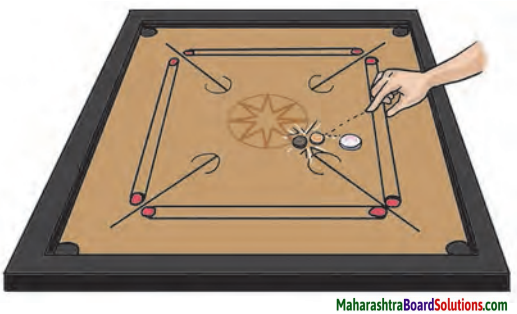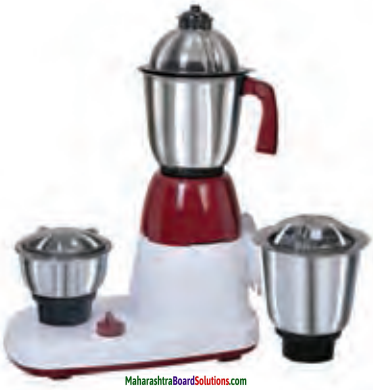Balbharti Maharashtra State Board Class 6 Science Solutions Chapter 10 Force and Types of Force Notes, Textbook Exercise Important Questions and Answers.

## Maharashtra State Board Class 6 Science Solutions Chapter 10 Force and Types of Force

Class 6 Science Chapter 10 Force and Types of Force Textbook Questions and Answers

1. Choose the term and fill in the blanks:

Question a.
……….. has to be applied to change the ……….. of a ……….. object.
(moving, direction, force)
force, direction, moving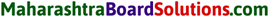Question b.
When an elephant drags a wooden log over the land, the forces that are applied on the log are …………., ………. and …………. .
(muscular force, mechanical force, gravitational force, frictional force)
muscular force, gravitationalforce, frictionalforce.

Question c.
A ball was set rolling on a large table. If its …….. has to be changed, a ……….. will have to be applied on it.
(force, motion, gravitation)
motion, force

Question d.
The force of friction always acts ……….. the motion.
(along, against)
against2. Match the following:

Question a.

 Column ‘A’ Column ‘B’ 1. An ox pulling a cart a. Magnetic force 2. Lifting heavy iron object with a crane b. Electrostatic force 3. Weighing with a spring balance c. Muscular force 4. Applying brakes to a bicycle. d. Gravitational force 5. Picking up pieces of paper with a plastic scale. e. Frictional force

 Column ‘A’ Column ‘B’ 1. An ox pulling a cart c. Muscular force 2. Lifting heavy iron object with a crane a. Magnetic force 3. Weighing with a spring balance d. Gravitational force 4. Applying brakes to a bicycle. e. Frictional force 5. Picking up pieces of paper with a plastic scale. b. Electrostatic force3. One or more forces are acting in the following examples. Name them.

Question a.
An object falling from a tall building …………. .
Gravitational force

Question b.
An aeroplane flying in the sky. …………. .
Mechanical force

Question c.
Sqeezing sugarcane juice with a squeezer. …………. .
Muscular or mechanical forceQuestion d.
Winnowing foodgrain …………. .
Muscular force, gravitational force

4. Explain in your own words giving one example each.
Muscular force, gravitational force, mechanical force, electrostatic force, the force of friction and magnetic force.

Question a.
Muscular force:
The force applied with the help of muscles is called muscular force.
e.g. When a bullock pulls the load of a cart with sugarcane, he uses the force of his muscles that is muscular force.

Question b.
Gravitational force:
The force applied by the earth to pull the objects towards itself is called gravitational force.
e.g. When a fruit falls from a tree, it is the gravitational force of the earth that pulls the fruit.Question c.
Mechanical force:
The force applied by means of a machine is called mechanical force. Some machines run by muscular force while some use electricity or fuel.
e.g.Washing machine, electric pump uses electricity to work.

Question d.
Electrostatic force:
Static electricity is produced on materials like rubber, plastic and ebonite due to friction. The force exerted by such electrically charged materials is called electrostatic force, e.g. When a plastic comb is rubbed against the dry hair, comb develops electrostatic charge.

Question e.
The force of friction:
When two surfaces rub against each other, a force of friction is produced. This force always acts against direction of motion, e.g. A ball rolling over a flat surface stops at certain distance due to friction between the ball and the surface.

Question f.
Magnetic force:
The force exerted by a magnet is called magnetic force.
e.g. In a pin holder, the magnet attracts the pins.5. Why?

Question a
Machines are oiled from time to time.
To reduce the friction between the parts of machines, they are oiled. This will help efficient working of machines.

Question b.
An object thrown upwards comes down after reaching a point.
An object is pulled by the gravitational force of the earth. Hence an object thrown upwards comes down after reaching at certain point.

Question c.
Powder is sprinkled on a carrom board.
When powder is sprinkled on a carrom board, friction between the coin and the board decreases so that the coin can be pocketed easily.Question d.
The ramp at a railway station has a rough surface.
The rough surface of the ramp increases the friction between feet and the surface. This helps us to walk on the ramp without skidding or falling.

6. In what way are we different?

Question a.
Muscular force and Mechanical force

 Muscular force Mechanical force 1. The force applied with the help of muscles is called muscular force. e.g. A boy kicking a football. 1. The force applied by means of machine is called mechanical force. e.g. An electric pump works on electricity. 2. It is created by our muscles. 2. It is created by electricity or fuel or muscular forceQuestion b.
A force of friction and Gravitational force

 Force of friction Gravitational force 1. When two surfaces rub against each other, the force of friction comes into force. 1. The force applied by the earth to pull an object towards itself, is called a gravitational force. 2. It comes to act between two surfaces. 2. It exists between two objects or an object and the earth.

Question a.
What are the things that can be done by applying force?
By applying force:

1. we can set an object in motion.
2. we can stop a moving object.
3. we can change the speed or direction or both of a moving object.
4. we can change the shape of an object.

Question b.
What is meant by weight?

1. The gravitational force acting on an object is called its weight.
2. Greater force must be applied to lift a greater weight.Question c.
Which machines run on muscular force?
The machines that run on muscular force are:

1. Bicycle
2. Hand cart
3. Boat
4. Cycle rickshaw
5. Stretching of a spring
6. Ball pen
7. Vegetable chopper
8. Sharpener
9. Pulley
10. Fruit peeler

8. Solve the following crossword puzzle.

Question a.
Solve the following crossword puzzle.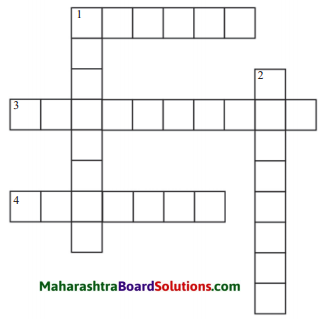Down:
1. ………. force is applied to push a scooter that has failed.
2. ………. force can be used to pick up scattered pins.

Across:
3. A ………. pulls iron nails towards itself.
4. ……….. force is used when farm is ploughed with a tractor.
5. It is due to the force of ………… that raindrops fall to the ground.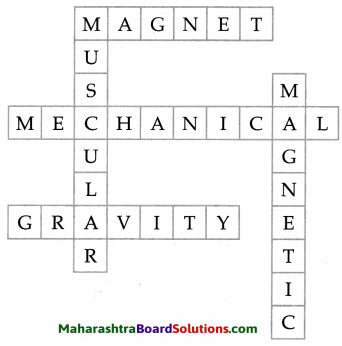Activity:

Question 1.

Question 2.
Make a list of such actions where many forces are applied simultaneously.

Class 6 Science Chapter 10 Force and Types of Force Important Questions and Answers

Choose the term and fill in the blanks:

Question 1.
When powder is sprinkled on a carrom board, friction ……….. .
(increases, decreases)
decreasesQuestion 2.
Electrostatic force is due to …………. .
(gravitation, friction)
friction

Question 3.
Fruits fall on the ground due to ………… force.
(mechanical, gravitational)
gravitational

Question 4.
Friction between two rough surfaces is …………. than between two smooth surfaces.
(greater, lesser)
greater

Question 5.
The sound created by a stone dropped in a bucket of water from 100 cm height is ………… than that dropped from 20 cm height.
(milder, louder)
louderQuestion 6.
A scooter has stopped in the middle of the road as the petrol tank has become empty. So Raju has to use …………. force to take it to petrol pump.
(mechanical, muscular)
muscular

One or more forces are acting in the following examples. Name them.

Question 1.
Muscular force

Question 2.
Raju rubs a peacock feather between pages of his notebook
Electrostatic forceQuestion 3.
When you rub the palm of your hands, you feel the heat on the palms
Frictional force

Question 4.
A blacksmith uses a hammer to beat the hot piece of iron
Muscular force

Question 5.
A cricketer hits the ball, but it stops just before the boundary line
Frictional force

Question 6.
We can walk on the road without falling down.
Frictional forceGive scientific reasons:

Question 1.
Magnet is used to lift heavy iron bar.
A magnet attracts the iron bar. As a result it is easier to lift the heavy iron bar using magnetic force.

Question 2.
An inflated balloon when rubbed against a silk cloth, pulls the hair on our skin.
When an inflated balloon is rubbed against a silk cloth, it develops electrostatic force due to friction. This force pulls the hair on our skin.

Question 3.
We are likely to slip when we walk on a oily or wet floor.
Wet or oily floor reduces the friction between our feet and surface. Hence we are likely to slip on wet or oily floor.

Distinguish between:

Question 1.
Force of friction and Electrostatic force

 Force of friction Electrostatic force 1. It exists between any two surfaces in contact. 1. It develops where certain objects are rubbed  against certain material. 2. No electric charge is developed, sometimes heat is developed. e.g. A brake is applied on a bicycle. 2. Electric charge is developed. e.g. Thermocol rubbed against silk cloth.Question 1.
Why do planets revolve round the sun in definite orbits?

1. The gravitational force operates between the sun and the planets in the solar system.
2. Hence, they revolve round the sun in definite orbits.

Question 2.
Which forces are acting upon an aeroplane taking off into the sky?
Aeroplane uses mechanical force, Pilot exerts muscular force. Frictional force between aeroplane and air, gravitational force due to weight of an aeroplane.

Question 3.
What happens to the iron nail when the magnet is taken away from it?

1. As we take the magnet away from the iron nail, it does not attract the iron nail as magnetic force decreases.
2. Magnet is unable to exert force if the nail is not placed in the range of the magnetic field.Question 4.
When brakes are applied while riding a bicycle, it stops after running a distance. Why?

1. When brakes are applied while riding a bicycle, frictional force comes to act between the tyres of the bicycle and the surface.
2. This force opposes the tendency of the bicycle to stop.
3. Hence, the bicycle has to overcome this force before it stops.

Can you tell?

Question 1.
What happens when you hold the two ends of a spring and pull them apart?
Its shape changes due to the force exerted by pull.

Question 2.
What happens when a blacksmith hammers a red hot piece of iron?
Shape of iron piece changes.

Observe and discuss:

Observe the figures given and name the force needed for the motion.

Question 1.
Boat with boatman.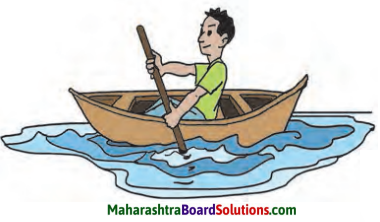Muscular forceQuestion 2.
Bullock cart.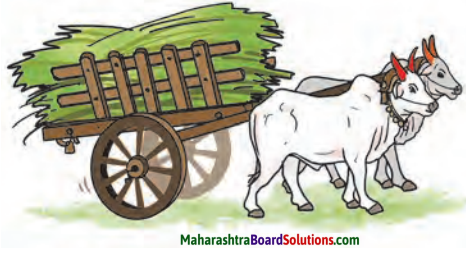Muscular force

Question 3.
A tractor.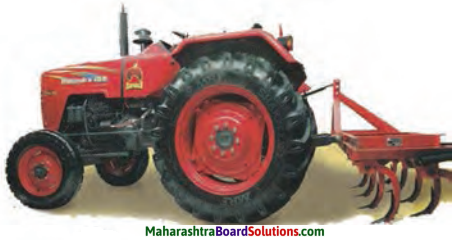Mechanical force

Question 4.
Sewing machine.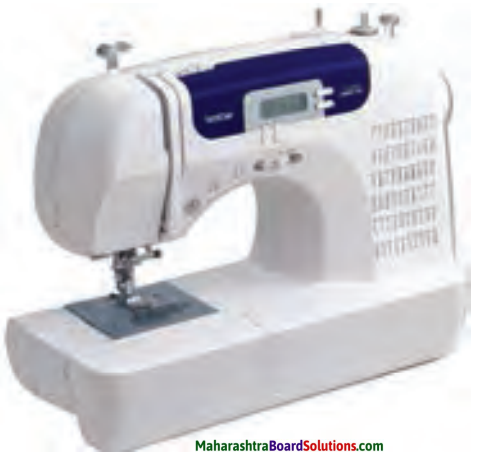Mechanical forceQuestion 5.
Fruit falling from tree.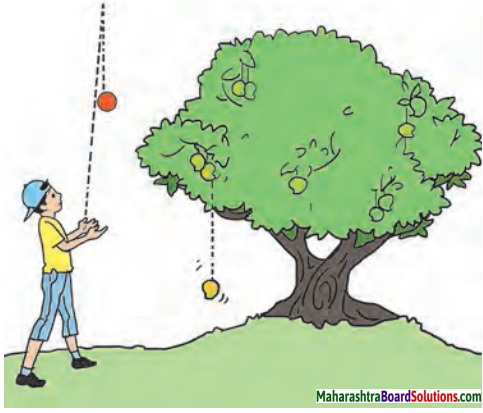Gravitational force

Question 6.
A man carrying heavy sac.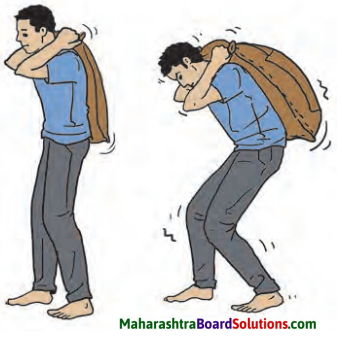Gravitational force

Question 7.
A pin holder.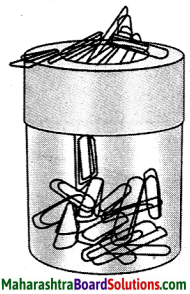Magnetic force

Question 8.
A ramp.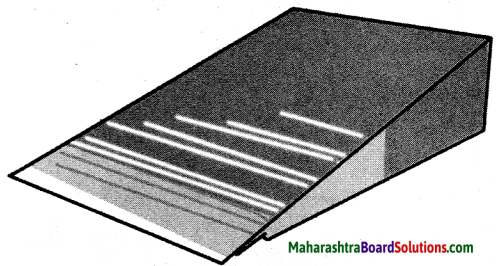Gravitational forceQuestion 9.
Carrom board.